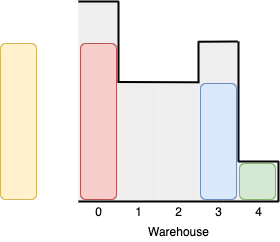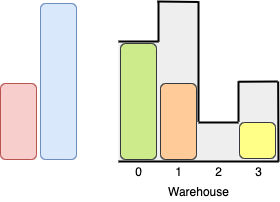Formatted question description: https://leetcode.ca/all/1564.html

# 1564. Put Boxes Into the Warehouse I

Medium

## Description

Given two arrays of positive integers boxes and warehouse representing the heights of some boxes of unit width, and the heights of n rooms in a warehouse, respectively. The warehouse’s rooms are labeled from 0 to n - 1 from left to right where warehouse[i] (0-indexed) is the height of the i-th room.

Boxes are put into the warehouse by the following rules:

• Boxes can’t be piled up.
• You can rearrange the order of the boxes.
• Boxes can only be pushed into the warehouse from left to right only.
• If the height of some room in the warehouse is less than the height of a box, then the box will be stopped before that room, so are the boxes behind it.

Return the maximum number of boxes you can put into the warehouse.

Example 1:Input: boxes = [4,3,4,1], warehouse = [5,3,3,4,1]

Output: 3

Explanation:We can first put the box of height 1 in room 4. Then we can put the box of height 3 in either of the 3 rooms 1, 2, or 3. Lastly, we can put one box of height 4 in room 0.

There is no way we can fit all 4 boxes in the warehouse.

Example 2:Input: boxes = [1,2,2,3,4], warehouse = [3,4,1,2] Output: 3

**Explanation: **Notice that it’s not possible to put the box of height 4 into the warehouse since it cannot pass the first room of height 3.

Also, for the last two rooms, 2 and 3, only boxes of height 1 can fit.

We can fit 3 boxes maximum as shown above. The yellow box can also be put in room 2 instead.

Swapping the orange and green boxes is also valid, or swapping one of them with the red box.

Example 3:

Input: boxes = [1,2,3], warehouse = [1,2,3,4]

Output: 1

Explanation: Since the first room in the warehouse is of height 1, we can only put boxes of height 1.

Example 4:

Input: boxes = [4,5,6], warehouse = [3,3,3,3,3]

Output: 0

Constraints:

• n == warehouse.length
• 1 <= boxes.length, warehouse.length <= 10^5
• 1 <= boxes[i], warehouse[i] <= 10^9

## Solution

For 0 <= i < n - 1, if warehouse[i] < warehouse[i + 1], then the maximum valid height of room warehouse[i + 1] is only warehouse[i]. Therefore, create a new array validWarehouse with length n, where validWarehouse = warehouse and validWarehouse[i] = Math.min(validWarehouse[i - 1], warehouse[i]) for 1 <= i < n.

Sort boxes. Loop over boxes in forward direction and loop over validWarehouse in backward direction. For each box, find the room with the minimum height that can contain the box, and put the box into the room. Since all the remaining boxes have equal height or greater height than the current box, only the rooms with equal height or greater height are considered for the remaining boxes. Repeat the process until all the boxes are put into the warehouse or all the valid rooms in the warehouse are used. Finally, return the number of boxes that are put into the warehouse.

class Solution {
public int maxBoxesInWarehouse(int[] boxes, int[] warehouse) {
int roomsCount = warehouse.length;
int[] validWarehouse = new int[roomsCount];
validWarehouse = warehouse;
for (int i = 1; i < roomsCount; i++)
validWarehouse[i] = Math.min(validWarehouse[i - 1], warehouse[i]);
int maxBoxes = 0;
Arrays.sort(boxes);
int boxesCount = boxes.length;
for (int i = 0, j = roomsCount - 1; i < boxesCount && j >= 0; i++) {
while (j >= 0 && boxes[i] > validWarehouse[j])
j--;
if (j >= 0 && boxes[i] <= validWarehouse[j]) {
maxBoxes++;
j--;
}
}
return maxBoxes;
}
}